International
Tables for
Crystallography
Volume B
Reciprocal space
Edited by U. Shmueli

International Tables for Crystallography (2006). Vol. B, ch. 5.1, pp. 534-535   | 1 | 2 |

## Section 5.1.2.1. Propagation equation

A. Authiera*

aLaboratoire de Minéralogie-Cristallographie, Université P. et M. Curie, 4 Place Jussieu, F-75252 Paris CEDEX 05, France
Correspondence e-mail: authier@lmcp.jussieu.fr

#### 5.1.2.1. Propagation equation

| top | pdf |

The wavefunction Ψ associated with an electron or a neutron beam is scalar while an electromagnetic wave is a vector wave. When propagating in a medium, these waves are solutions of a propagation equation. For electrons and neutrons, this is Schrödinger's equation, which can be rewritten as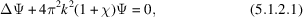where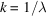is the wavenumber in a vacuum,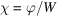(ϕ is the potential in the crystal and W is the accelerating voltage) in the case of electron diffraction and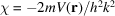[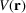is the Fermi pseudo-potential and h is Planck's constant] in the case of neutron diffraction. The dynamical theory of electron diffraction is treated in Chapter 5.2[note that a different convention is used in Chapter 5.2for the scalar wavenumber:; compare, for example, equation (5.2.2.1) and its equivalent, equation (5.1.2.1)] and the dynamical theory of neutron diffraction is treated in Chapter 5.3.

In the case of X-rays, the propagation equation is deduced from Maxwell's equations after neglecting the interaction with protons. Following von Laue (1931, 1960), it is assumed that the positive charge of the nuclei is distributed in such a way that the medium is everywhere locally neutral and that there is no current. As a first approximation, magnetic interaction, which is very weak, is not taken into account in this review. The propagation equation is derived in Section A5.1.1.2of the Appendix. Expressed in terms of the local electric displacement,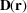, it is given for monochromatic waves by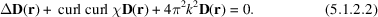The interaction of X-rays with matter is characterized in equation (5.1.2.2)by the parameter χ, which is the dielectric susceptibility. It is classically related to the electron densityby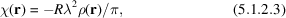where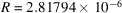nm is the classical radius of the electron [see equation (A5.1.1.2)in Section A5.1.1.2of the Appendix].

The dielectric susceptibility, being proportional to the electron density, is triply periodic in a crystal. It can therefore be expanded in Fourier series: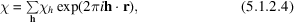where h is a reciprocal-lattice vector and the summation is extended over all reciprocal-lattice vectors. The sign convention adopted here for Fourier expansions of periodic functions is the standard crystallographic sign convention defined in Section 2.5.2.3. The relative orientations of wavevectors and reciprocal-lattice vectors are defined in Fig. 5.1.2.1, which represents schematically a Bragg reflection in direct and reciprocal space (Figs. 5.1.2.1aand 5.1.2.1b, respectively).Figure 5.1.2.1 | top | pdf |Bragg reflection. (a) Direct space. Bragg reflection of a wave of wavevector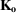incident on a set of lattice planes of spacing d. The reflected wavevector is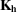. Bragg's law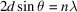can also be written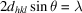, where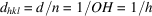is the inverse of the length of the corresponding reciprocal-lattice vector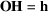(see part b). (b) Reciprocal space. P is the tie point of the wavefield consisting of the incident wave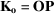and the reflected wave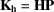. Note that the wavevectors are oriented towards the tie point.

The coefficients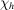of the Fourier expansion of the dielectric susceptibility are related to the usual structure factor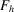by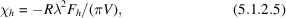where V is the volume of the unit cell and the structure factor is given by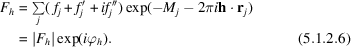is the form factor of atom j,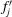and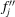are the dispersion corrections [see, for instance, IT C, Section 4.2.6] and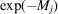is the Debye–Waller factor. The summation is over all the atoms in the unit cell. The phaseof the structure factor depends of course on the choice of origin of the unit cell. The Fourier coefficientsare dimensionless. Their order of magnitude varies fromto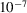depending on the wavelength and the structure factor. For example,is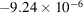for the 220 reflection of silicon for Curadiation.

In an absorbing crystal, absorption is taken into account phenomenologically through the imaginary parts of the index of refraction and of the wavevectors. The dielectric susceptibility is written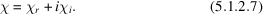The real and imaginary parts of the susceptibility are triply periodic in a crystalline medium and can be expanded in a Fourier series,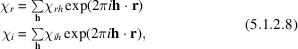where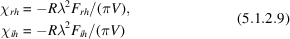and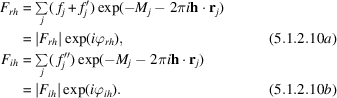It is important to note that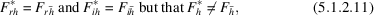where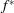is the imaginary conjugate of f.

The index of refraction of the medium for X-rays is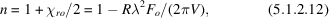where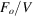is the number of electrons per unit volume. This index is very slightly smaller than one. It is for this reason that specular reflection of X-rays takes place at grazing angles. From the value of the critical angle,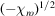, the electron densityof a material can be determined.

The linear absorption coefficient is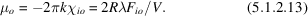For example, it is 143.2 cm−1 for silicon and Cu Kα radiation.

### References

Laue, M. von (1931). Die dynamische Theorie der Röntgenstrahl interferenzen in neuer Form. Ergeb. Exakten Naturwiss. 10, 133–158.Google Scholar
Laue, M. von (1960). Röntgenstrahl-Interferenzen. Frankfurt am Main: Akademische Verlagsgesellschaft.Google Scholar